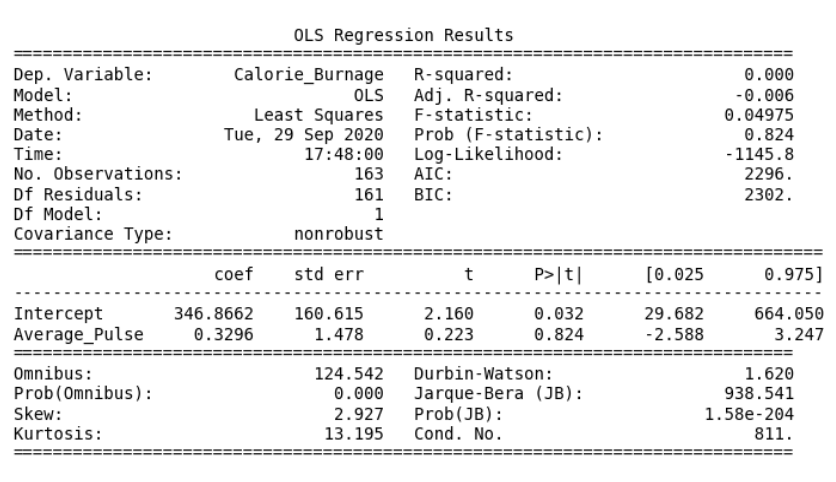# Data Science - Regression Table

## Regression Table

The output from linear regression can be summarized in a regression table.

The content of the table includes:

• Coefficients of the linear regression function
• Regression statistics
• Statistics of the coefficients from the linear regression function
• Other information that we will not cover in this module

## Regression Table with Average_Pulse as Explanatory Variable## Create a Linear Regression Table in Python

Here is how to create a linear regression table in Python:

### Example

import pandas as pd
import statsmodels.formula.api as smf